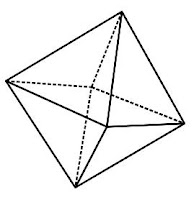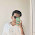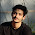## Sunday, 29 July 2012

### CBSE Class 9 - Maths - Ch6 - Lines and Angles (Set-2)Octahedron
Lines and Angles: Q & A

Q1: Prove that two lines which are both parallel  to the same line, are parallel to each other.

Given: Three lines l, m, n in a plane such that l || m and m || n.

To prove: l || n

Proof:  Suppose line l is not parallel to line n. Then l, n will intersect at some unique point, say at P.

⇒ P lies on l but does not lie on m, since l || m.

∴ Through point P outside m, there are two lines ( l and n ) and both are parallel to line m.  This is not possible (violates Parallel Axiom).

∴ our assumption is wrong.

Hence l || n.

Q2: Prove that, if P is a point which divides the line segment AB in the ratio m:n internally, then P is unique.

Suppose point P divides segment AB in the ratio m:n internally. Let us assume P is not unique.
⇒ There is another point P1 which also divides the segment in ratio m:n internally.
∴  AP/PB = m:n               and      AP1/P1B = m/n
∴  nAP = mPB                 and      nAP1 =mP1B
nAP = m(AB - AP)     and      nAP1 = m( AB - AP1)
⇒ nAP = mAB - mAP     and      nAP1 = mAB - mAP1
⇒ (m+n) AP = mAB       and       (m + n)AP1 = mAB
⇒ AP = mAB/(m+n)       and       AP1 = mAB/(m+n)
∴ AP  =   AP1
∴ P and P1 are the same point, which contradicts our assumption.
Hence P is unique.

Q3: How many least number of distinct points determine a unique line?

Q4: In how many point two distinct lines can intersect?

Q5: In how many points two distinct planes can intersect?

Answer:  Infinite number of points.

Q6: If B lies between A and C  and AC = 8, BC = 3. What is AB and AB2 ?

Answer: Since B lies between A and C,
∴  AB + BC = AC
BC = 3 and AC = 8
AB = AC - BC = 8 - 3 = 5
AB2 = 5 ✕ 5 = 25

Q7:  An angle is 14° more than its complement. Find the angle?

Answer: Let the angle be p°.
Its complementary angle = 90° - p°
As per the given information,
p°  = (90° - p°) + 14°
⇒  p° + p° = 90° + 14°
⇒  2p° = 104°
⇒  p° = 52°

Q8: What angle is equal to its supplement?

Let the angle be y°.
∴ The other angle is = 180 - y
Since two angle are equal,
⇒ y = 180 - y
⇒ 2y = 180
⇒ y = 90°1.2.3.